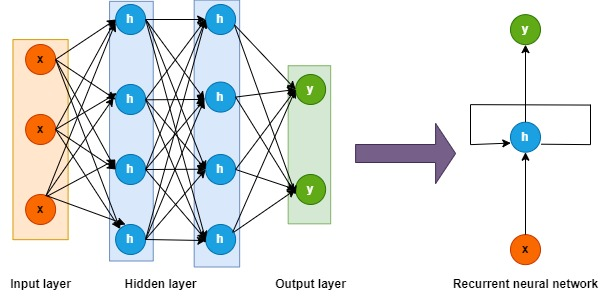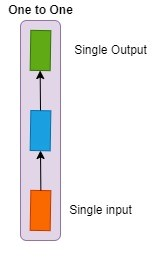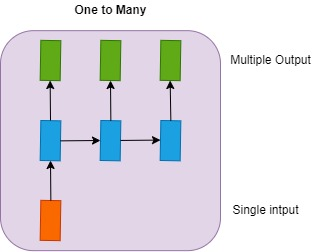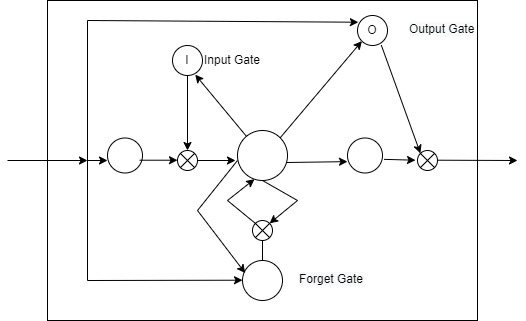##### Introduction

Neutral Networks is one of favored algorithm as it is faster and accurate. Therefore, it is extremely important to know what a Neural Network is, how it is constructed, and what its capabilities and limitations are.

##### What Is a Neural Network?

With the use of large amounts of data and complex algorithms, the Neural Network simulates the structure and function of the brain.

Let us take an example of dog breeds to illustrate

• As input images of different dogs are fed.
• Using those image pixels in the hidden layers, Feature extraction is then performed.
• This layer produces a response to determine if it’s a Rottweiler or Husky
• This type of network does not require that past output be memorized.

Different business problems can be solved by neural networks. Here are a few examples.

• Regression and classification problems can be solved with a feed-forward neural network.
• Object detection and image classification are performed using convolutional neural networks.
• Cancer detection using Deep Belief Networks in healthcare sectors.
• Speech and voice recognition, time series prediction, and natural language processing are all utilized by RNN.
##### Explain Recurrent Neural Network (RNN)

In order to predict the output of a layer, RNN saves the output of a particular layer and feeds that back to the input.

A Feed-Forward Neural Network can be converted to a Recurrent Neural Network by following these steps:Recurrent neural networks are formed by combining the nodes in different layers.“X” represents the input layer, “h” represents the hidden layer, and “y” represents the output layer. This model’s output is improved by modifying network parameters A, B, and C. This model’s input is a combination of input at x(t) and x(t-1). Whenever possible, output from the network is retrieved to improve it.

##### Why Recurrent Neural Networks?

A few problems with feed-forward neural networks led to the creation of RNNs:

• Sequential data cannot be handled
• Only takes into account the current input
• Having trouble remembering previous inputs

RNNs provide a solution to these issues. It is possible to apply RNNs to sequential data by accepting the current input data, as well as previously received inputs. The internal memory of RNNs allows them to remember previous inputs.

##### Explain working of Recurrent Neural Networks

The information is cycled through a loop to the middle hidden layer in Recurrent Neural Networks.The input layer ‘x’ receives the input to the neural network and processes it before passing it on to the middle layer. Layers ‘h’ can be comprised of multiple hidden layers, with separate activation functions, weights, and biases each. You can use a recurrent neural network if the parameters of the hidden layers of a neural network are not affected by the previous layer, i.e. the neural network has no memory.

By standardizing the different activation functions, weights, and biases in the Recurrent Neural Network, the hidden layers will have the same parameters. Then, instead of creating multiple hidden layers, it will create one and loop over it as many times as required.

##### Feed-Forward Neural Networks vs Recurrent Neural Networks

Information can flow through a feed-forward neural network only in one direction, from the input nodes through the hidden layers, and from the hidden to the output nodes. The network has no loops or cycles.

Here is a simplified representation of a feed-forward neural network:Based on the current input, feed-forward neural networks make decisions. The past data isn’t memorized, nor is the future incorporated. In general regression and classification problems, feed-forward neural networks are used.

• #### Image Captioning

By analyzing the activities present in an image, RNNs caption images

eg: Hitting a bottle hanging.

• #### Time Series Prediction

A RNN can be used to solve any time series problem, such as predicting stock prices in a given month.

• #### Natural Language Processing

Sentiment analysis can be performed by using RNNs for Natural Language Processing (NLP)

• #### Machine Translation

RNNs can translate inputs in one language into different languages as outputs from an input in a language.

##### Explain the types of Recurrent Neural Networks

Recurrent neural networks can be divided into four types:

1. One to One
2. One to Many
3. Many to One
4. Many to Many
##### One to One RNN

It is known as a Vanilla Neural Network. Generally, it is used when there is only one input and one output in a machine learning problem##### One to Many RNN

A single input and multiple outputs are present in this type of neural network.

e.g. image caption##### Many to One RNN

One output is generated by this RNN from a sequence of inputs. As an example of this, sentiment analysis can be used to classify a given sentence as positive or negative.##### Many to Many RNN

An RNN generates a sequence of outputs from a sequence of inputs. A typical example of this is machine translation.##### Issues with Standard RNNs

With recurrent neural networks, you can model time-dependent and sequential data problems, such as forecasting stock markets, translating texts, and generating texts. The gradient problem, however, makes RNN hard to train.

The vanishing gradient problem affects RNNs. In RNNs, gradients are used to carry information, and when the gradient becomes too small, parameter updates become insignificant. As a consequence, long data sequences are difficult to learn.

An Exploding Gradient is when a neural network’s slope grows exponentially instead of decaying. In the training process, large error gradients accumulate, which leads to a very large update to the neural network model weights.

The main issues with gradient problems are long training times, poor performance, and bad accuracy.

##### Backpropagation through Time

As a machine learning algorithm, backpropagation is widely known as a workhorse. Backpropagation is a method for computing a neural network’s gradient with respect to its weights. In order to find the partial derivative of the errors based on the weights, the algorithm works backwards through the layers of gradients. This results in a lower error margin during training. Recurrent neural networks that process time series data are back propagated through time by using the Backpropagation algorithm.

RNNs are typically fed one input at a time, and one output is obtained. In backpropagation, however, you use both current and previous inputs as inputs. This is referred to as a time step, and one time step will contain many time series data points entering simultaneously into an RNN. The output is used to calculate and accumulate the errors after the neural network has trained on a given time set. As a result, the network is rolled back up and weights are recalculated and updated while keeping the errors in mind.

##### Long Short-Term Memory Networks

Long short-term memory (LSTM) networks extend the memory of RNNs. A RNN is constructed using LSTMs as the building blocks. By assigning weights to data, LSTMs help RNNs either let in new information, forget information, or give it enough importance to influence them.An LSTM is used to build layers of a RNN, often called an LSTM network.

RNNs can remember input over a long period of time using LSTMs. A LSTM is a memory that stores information, similar to a computer’s memory. Data can be read, written, or deleted from the LSTM memory.It can be viewed as a gated memory, with gated meaning the memory decides whether or not to store or delete information (i.e., if it opens or closes the gates), based on the importance it assigns to the information. Priority is assigned through weights, which are also learned by the algorithm. Over time, it learns what information is important and what is not.

Three gates make up an LSTM: input, forget, and output gates. The gates decide whether new input should be allowed in (input gate), whether it should be deleted because it isn’t important (forget gate), or whether it should impact output at the current time step (output gate). A RNN with three gates is illustrated below:An LSTM’s gates are analog, meaning they range from 0 to 1. Due to their analog nature, they can perform backpropagation.

The LSTM solves the problem of vanishing gradients by keeping gradients steep enough, keeping training time short and accuracy high.

Thank you very much for reading . Hope you enjoyed the article!